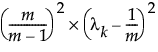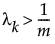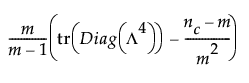Publication date: 05/24/2021

The usual principal inertias of a Burt table constructed from m categorical variables in MCA are the eigenvalues λk from Λ2. These inertias provide a pessimistic indication of fit. Benzécri (1979) proposed the following inertia adjustment; it is also described by Greenacre (1984, p. 145):forThis adjustment computes the percent of adjusted inertia relative to the sum of the adjusted inertias for all inertias greater than 1/m.

Greenacre (1984, p. 156) argues that the Benzécri adjustment overestimates the quality of fit. Greenacre proposes instead to compute the percentage of adjusted inertia relative to:for all inertias greater than 1/m, where tr(Diag(Λ4)) is the sum of squared inertias and nc is the total number of categories across the m variables.